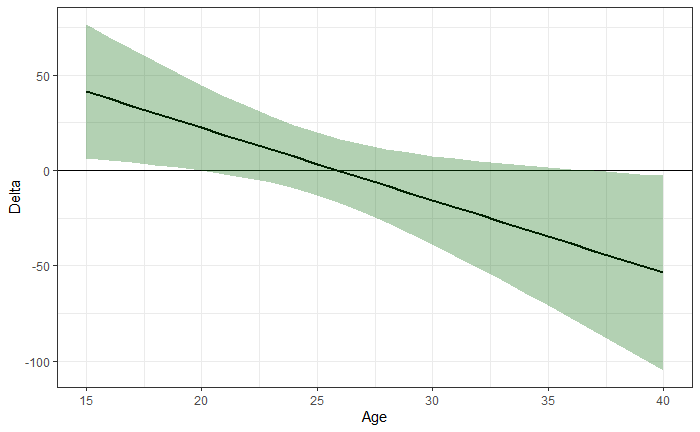# “Effect or Treatment Heterogeneity? Policy Evaluation with Aggregated and Disaggregated Treatments”

## Scenario 1: Smoking and birth weight

This Notebook replicates the results of section 8.1 using a publicly available random subsample of the data (GitHub repository of Matias Cattaneo) with 5000 instead of roughly 500,000 observations. The notebook should help to …

• show the underlying code for researchers interested in replicating the analysis or in adapting it to new datasets

• show that the patterns are similar even with 100 times less data, although they are of course much more noisy

The explanations throughout the notebook are kept short. Please see the paper for more details regarding the decomposition parameters and their estimation.

Click on the “Code” button on the top right of this notebook to “Hide All Code” and see only the results or “Download Rmd” to extract the underlying code.

Running the analyses in this notebook took roughly two hours on a SWITCHengine with eight cores and 32 GB RAM.

## Data preparation

# Create main variables

library(foreign)
library(causalDML)
library(tidyverse)

# Set wd and load paper specific functions

# Set seed
set.seed(1234)

data = read.dta("C_2010_JOE-dataRS5K.dta")

Now prepare outcome, effective treatment, and confounders as well as the two heterogeneity variables ethnicity and age:

# Create main variables
Y = as.vector(data[,1])
T = as.vector(data[,2])
X = as.matrix(data[,4:53])

## Define heterogeneity variables
# Create categorical variable of ethnicity
ethnic = rep("Other",length(Y))
ethnic[X[,2] == 1] = "White"
ethnic[X[,3] == 1] = "Black"
ethnic[X[,4] == 1] = "Hispanic"
ethnic_oh = model.matrix(~0 + ethnic) # One hot coded dummy matrix
colnames(ethnic_oh) = c("Black","Hispanic","Other","White")

# Age
age = X[,44]

# Decomposition

## Double ML for the effective treatment

The implementation is based on the causalDML package that estimates the average potential outcomes and average treatment effects for the multivalued effective treatment. The nuisance parameters are estimated using an ensemble of methods, which first needs to be initialized. Unlike in the paper with 500k observations where two-fold cross-fitting and -validation is applied, we use 5-folds each with the 5k sample here. The rest is identical.

summary(ate$APO)   APO SE 0 3401.296 9.139818 1 3223.077 41.514047 2 3171.331 39.599396 3 3241.900 93.914800 4 3145.123 37.008544 5 3004.499 115.022326 summary(ate$ATE)
           ATE       SE       t         p
1 - 0 -178.219   42.382 -4.2051 2.655e-05 ***
2 - 0 -229.965   40.458 -5.6841 1.390e-08 ***
3 - 0 -159.395   94.280 -1.6907 0.0909643 .
4 - 0 -256.173   37.995 -6.7423 1.736e-11 ***
5 - 0 -396.797  115.357 -3.4397 0.0005871 ***
2 - 1  -51.746   57.342 -0.9024 0.3668819
3 - 1   18.823  102.655  0.1834 0.8545183
4 - 1  -77.954   55.565 -1.4029 0.1606990
5 - 1 -218.578  122.276 -1.7876 0.0739043 .
3 - 2   70.570  101.865  0.6928 0.4884830
4 - 2  -26.208   54.152 -0.4840 0.6284312
5 - 2 -166.832  121.637 -1.3716 0.1702647
4 - 3  -96.778  100.883 -0.9593 0.3374499
5 - 3 -237.401  148.518 -1.5985 0.1100013
5 - 4 -140.624  120.830 -1.1638 0.2445530
---
Signif. codes:  0 ‘***’ 0.001 ‘**’ 0.01 ‘*’ 0.05 ‘.’ 0.1 ‘ ’ 1
plot(ate$APO,label=c("None","1-5 cigs","6-10 cigs","11-15 cigs","16-20 cigs","> 20 cigs"))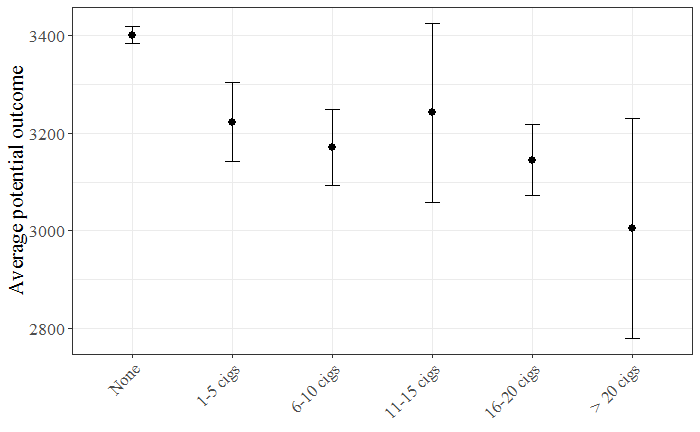## Decomposition The decomposition reuses the nuisance parameters and doubly robust scores that are stored in the object created by the causalDML function. ### Ethnicity First, we look at the discrete heterogeneity variable ethnicity and replicate the results of Figure 3a): ## Decomposition for each subgroup # Regression without constant decomp_ethnic_woc = HK_decomposition(ate$APO,ethnic_oh,intercept=FALSE)
summary(decomp_ethnic_woc)
nATE:
t test of coefficients:

Estimate Std. Error  t value  Pr(>|t|)
zBlack    -131.178     55.934  -2.3452 0.0190551 *
zHispanic -208.996     61.186  -3.4158 0.0006411 ***
zOther     -69.908     74.544  -0.9378 0.3483862
zWhite    -254.174     23.634 -10.7544 < 2.2e-16 ***
---
Signif. codes:  0 ‘***’ 0.001 ‘**’ 0.01 ‘*’ 0.05 ‘.’ 0.1 ‘ ’ 1

rATE:
t test of coefficients:

Estimate Std. Error t value  Pr(>|t|)
zBlack    -164.529     65.902 -2.4966  0.012573 *
zHispanic -240.730     76.136 -3.1618  0.001577 **
zOther     -69.137     78.367 -0.8822  0.377702
zWhite    -243.488     27.155 -8.9667 < 2.2e-16 ***
---
Signif. codes:  0 ‘***’ 0.001 ‘**’ 0.01 ‘*’ 0.05 ‘.’ 0.1 ‘ ’ 1

Delta:
t test of coefficients:

Estimate Std. Error t value Pr(>|t|)
zBlack     33.35066   23.93921  1.3931   0.1636
zHispanic  31.73400   25.44819  1.2470   0.2125
zOther     -0.77172   14.97663 -0.0515   0.9589
zWhite    -10.68540    9.91488 -1.0777   0.2812
plot(decomp_ethnic_woc)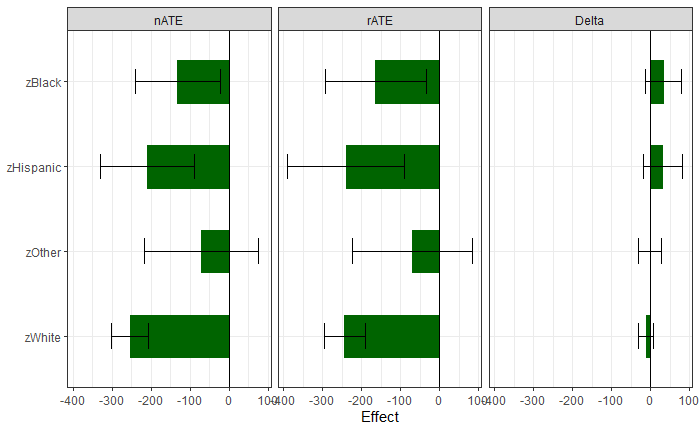and the results of Figure 3b):

# With white mothers as reference group
decomp_ethnic_wc = HK_decomposition(ate\$APO,ethnic_oh[,-4],intercept=TRUE)
summary(decomp_ethnic_wc)
nATE:
t test of coefficients:

Estimate Std. Error  t value Pr(>|t|)
(Intercept) -254.174     23.634 -10.7544  < 2e-16 ***
zBlack       122.996     60.723   2.0255  0.04287 *
zHispanic     45.178     65.592   0.6888  0.49100
zOther       184.265     78.201   2.3563  0.01850 *
---
Signif. codes:  0 ‘***’ 0.001 ‘**’ 0.01 ‘*’ 0.05 ‘.’ 0.1 ‘ ’ 1

rATE:
t test of coefficients:

Estimate Std. Error t value Pr(>|t|)
(Intercept) -243.4883    27.1548 -8.9667  < 2e-16 ***
zBlack        78.9596    71.2775  1.1078  0.26801
zHispanic      2.7586    80.8336  0.0341  0.97278
zOther       174.3517    82.9381  2.1022  0.03559 *
---
Signif. codes:  0 ‘***’ 0.001 ‘**’ 0.01 ‘*’ 0.05 ‘.’ 0.1 ‘ ’ 1

Delta:
t test of coefficients:

Estimate Std. Error t value Pr(>|t|)
(Intercept) -10.6854     9.9149 -1.0777  0.28121
zBlack       44.0361    25.9112  1.6995  0.08929 .
zHispanic    42.4194    27.3115  1.5532  0.12045
zOther        9.9137    17.9612  0.5520  0.58101
---
Signif. codes:  0 ‘***’ 0.001 ‘**’ 0.01 ‘*’ 0.05 ‘.’ 0.1 ‘ ’ 1
plot(decomp_ethnic_wc)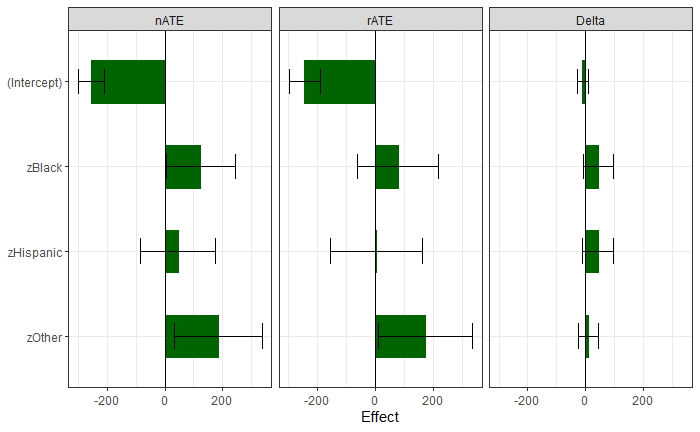### Age

Now consider the continuous heterogeneity variable age and estimate the heterogeneity in the decomposition parameters using B-splines as implemented in the crs package. We focus on mothers between 15 and 40 years old and trim the 59 younger or older mothers to avoid extreme behaviour at the boundaries.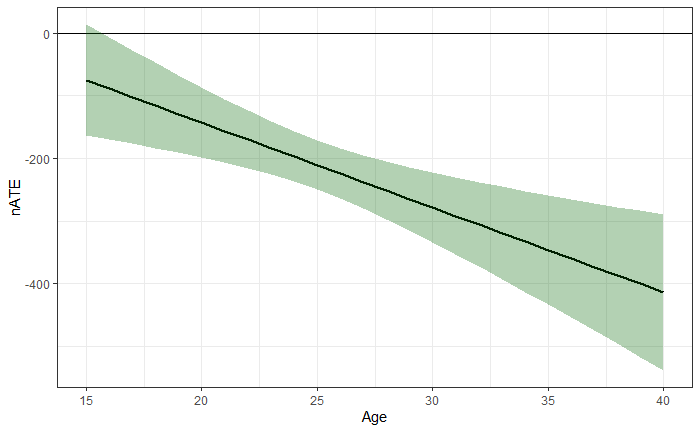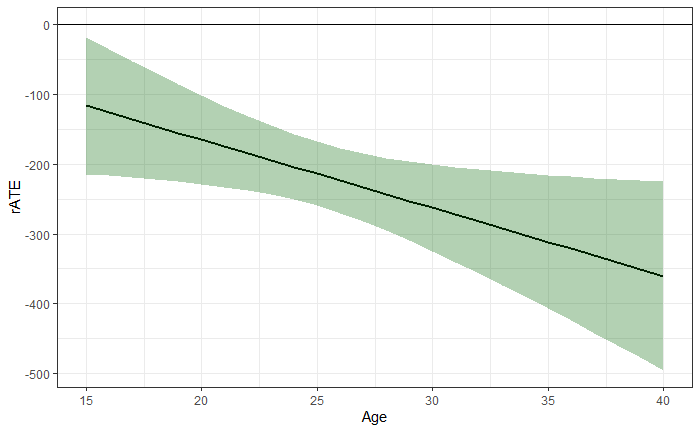# Plot resulting lines
pred_nATE = predict(decomp_age_trim, param = "nATE")
data.frame(z = age[sub],cate = pred_nATE[,1],
cilow = pred_nATE[,1] - 1.96 * pred_nATE[,2],
ciup = pred_nATE[,1] + 1.96 * pred_nATE[,2]) %>%
ggplot(mapping = aes(x = z, y = cate)) +
geom_line(size = .8) + geom_hline(yintercept=0) +
geom_ribbon(aes(ymin = cilow,max = ciup),alpha=0.3,fill="darkgreen") +
theme_bw() + ylab("nATE") + xlab("Age")

pred_rATE = predict(decomp_age_trim, param = "rATE")
data.frame(z = age[sub],cate = pred_rATE[,1],
cilow = pred_rATE[,1] - 1.96 * pred_rATE[,2],
ciup = pred_rATE[,1] + 1.96 * pred_rATE[,2]) %>%
ggplot(mapping = aes(x = z, y = cate)) +
geom_line(size = .8) + geom_hline(yintercept=0) +
geom_ribbon(aes(ymin = cilow,max = ciup),alpha=0.3,fill="darkgreen") +
theme_bw() + ylab("rATE") + xlab("Age")

pred_Delta = predict(decomp_age_trim, param = "Delta")
data.frame(z = age[sub],cate = pred_Delta[,1],
cilow = pred_Delta[,1] - 1.96 * pred_Delta[,2],
ciup = pred_Delta[,1] + 1.96 * pred_Delta[,2]) %>%
ggplot(mapping = aes(x = z, y = cate)) +
geom_line(size = .8) + geom_hline(yintercept=0) +
geom_ribbon(aes(ymin = cilow,max = ciup),alpha=0.3,fill="darkgreen") +
theme_bw() + ylab("Delta") + xlab("Age")